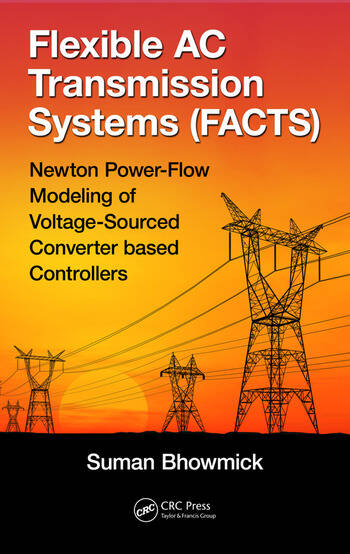# Flexible AC Transmission Systems (FACTS): Newton Power-Flow Modeling of Voltage-Sourced Converter-Based Controllers

## 1st Edition

Suman Bhowmick

CRC Press
Published May 10, 2016
Reference - 291 Pages - 111 B/W Illustrations
ISBN 9781498756198 - CAT# K27513

USD\$165.00

FREE Standard Shipping!

## Preview

### Summary

Flexible AC Transmission Systems (FACTS): Newton Power-Flow Modeling of Voltage-Sourced Converter-Based Controllers introduces different voltage-sourced converter (VSC)-based FACTS controllers and VSC-based high-voltage direct current (VSC-HVDC) systems and their working principles, explaining how FACTS controllers exchange real and reactive power with systems.

Subsequently, the book:

• Describes the Newton–Raphson method and its application for solving the power-flow problem
• Presents the Newton power-flow modeling of the static synchronous series compensator (SSSC), unified power-flow controller (UPFC), interline power-flow controller (IPFC), generalized unified power-flow controller (GUPFC), and static synchronous compensator (STATCOM), accommodating the practical device constraint limits (because of the unique modeling strategy, the existing Newton power-flow codes can be reused)
• Develops a unified Newton power-flow model of AC systems incorporating multiterminal VSC-HVDC systems with pulse-width modulation (PWM) control schemes, directly yielding the VSC modulation indices from the power-flow solution
• Provides numerous case studies for validation of Newton power-flow models, elaborating on the occurrences and checking of unrealistic power-flow solutions in isolated cases
• Includes detailed derivations of all the difficult formulae as well as solved problems on typical VSC-based FACTS controllers

Flexible AC Transmission Systems (FACTS): Newton Power-Flow Modeling of Voltage-Sourced Converter-Based Controllers assumes at least an undergraduate-level understanding of engineering mathematics, network analysis, electrical machines, electrical power systems, and power electronics. Thus, the book provides a valuable reference for practitioners as well as senior-undergraduate and graduate students in electrical engineering and electrical power systems.